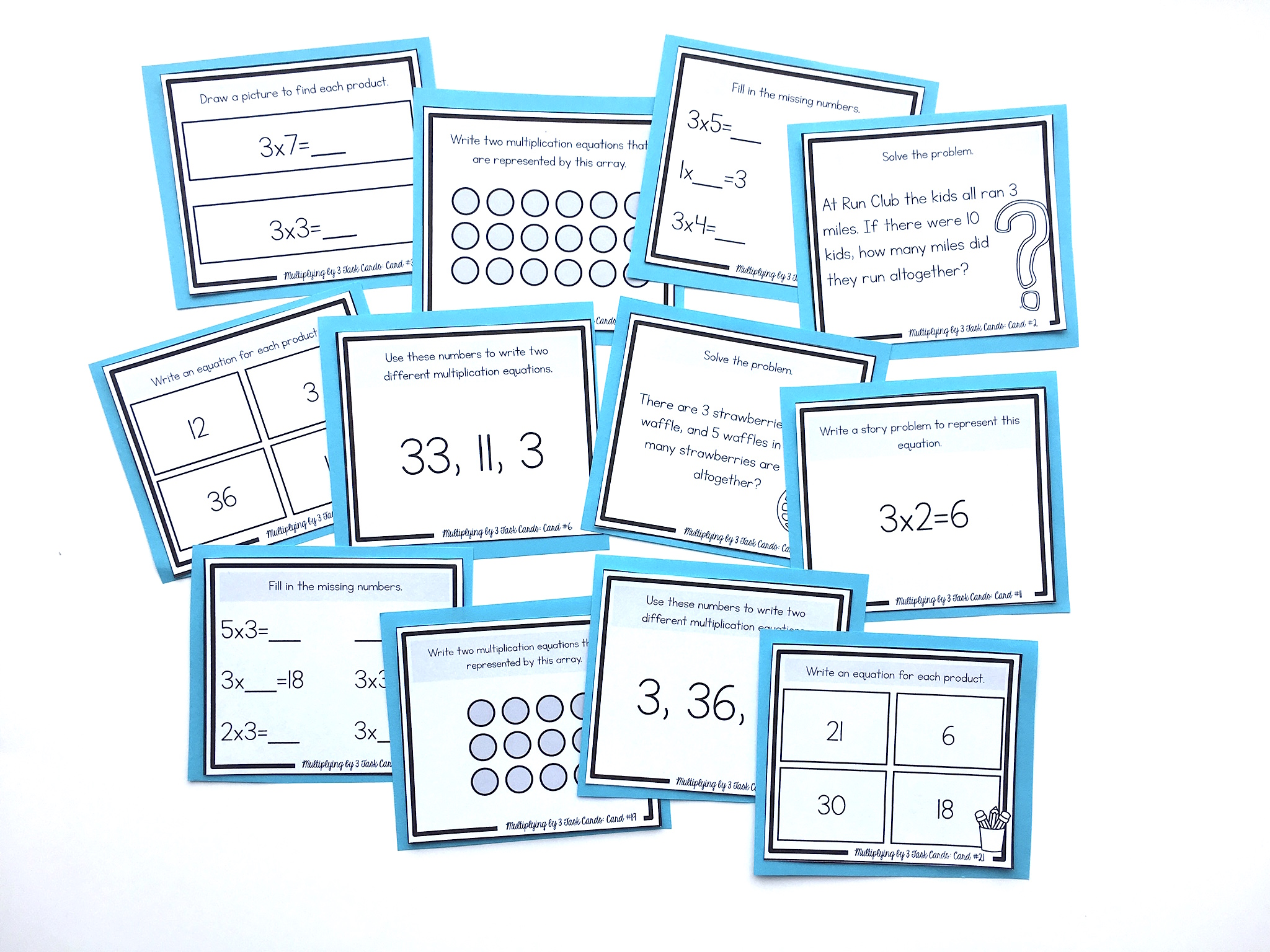# How to Teach the 3’s Multiplication FactsThe 3’s multiplication facts use students’ knowledge of the doubles addition facts. Students can think of a 3’s equation as “the double plus one more group.”

For example, to solve the equation 3×4, think, “the double of 4 plus one more group of 4,” which equals 12.

For 3×9, think, “the double of 9 plus one more group of 9,” to make 27.

It’s also very important to reinforce the commutative property.

This means that the order of factors does not change the product. For example, if students are faced with an equation like 5×3, they can still use this strategy since 3 is one of the factors. In this case, they can think, “the double of 5 plus one more group of 5,” to make 15.

NEXT STEPS

Reinforce the 3’s multiplication facts with this set of task cards. Students will learn conceptually through problem-solving, using arrays, strategic thinking, finding missing numbers, skip-counting, picture representations, and more:OR FIND THE FULL BUNDLE OF MULTIPLICATION TASK CARDS HERE.